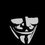# What is wrong with this proof?

i didn't get the mistake in this proof: 2+2=5.Not my proof. Found it on Facebook.textNote by Priyankar Kumar
6 years, 10 months ago

This discussion board is a place to discuss our Daily Challenges and the math and science related to those challenges. Explanations are more than just a solution — they should explain the steps and thinking strategies that you used to obtain the solution. Comments should further the discussion of math and science.

When posting on Brilliant:

• Use the emojis to react to an explanation, whether you're congratulating a job well done , or just really confused .
• Ask specific questions about the challenge or the steps in somebody's explanation. Well-posed questions can add a lot to the discussion, but posting "I don't understand!" doesn't help anyone.
• Try to contribute something new to the discussion, whether it is an extension, generalization or other idea related to the challenge.

MarkdownAppears as
*italics* or _italics_ italics
**bold** or __bold__ bold
- bulleted- list
• bulleted
• list
1. numbered2. list
1. numbered
2. list
Note: you must add a full line of space before and after lists for them to show up correctly
paragraph 1paragraph 2

paragraph 1

paragraph 2

[example link](https://brilliant.org)example link
> This is a quote
This is a quote
    # I indented these lines
# 4 spaces, and now they show
# up as a code block.

print "hello world"
# I indented these lines
# 4 spaces, and now they show
# up as a code block.

print "hello world"
MathAppears as
Remember to wrap math in $$ ... $$ or $ ... $ to ensure proper formatting.
2 \times 3 $2 \times 3$
2^{34} $2^{34}$
a_{i-1} $a_{i-1}$
\frac{2}{3} $\frac{2}{3}$
\sqrt{2} $\sqrt{2}$
\sum_{i=1}^3 $\sum_{i=1}^3$
\sin \theta $\sin \theta$
\boxed{123} $\boxed{123}$

Sort by:

$\sqrt{(4 - \frac{9}{2}) ^{2}}$ is not equal to $4 - \frac{9}{2}$

- 6 years, 10 months ago

thanks

- 6 years, 10 months ago

$\sqrt x^2 = |x|$ not $x$.

- 6 years, 10 months ago

You cant make a negative number positive by just squaring it and taking root. I'm talking about 2nd step, (4-(9/2))

- 6 years, 10 months ago

thanks

- 6 years, 10 months ago

$(4-\frac{9}{2})=-\frac{1}{2}$

but,

$(5-\frac{9}{2})=\frac{1}{2}$

in this proof we wrote--

$2+2=4-\frac{9}{2}+\frac{9}{2}...........step (i)$

$=\sqrt{(5-\frac{9}{2})^2}+\frac{9}{2}..........step(viii)$

$=5-\frac{9}{2}+\frac{9}{2}..........step(ix)$

so,what we actually doing is

$-\frac{1}{2}+\frac{9}{2}=\frac{1}{2}+\frac{9}{2}$..............consider step (i) and (ix)

this is where we made mistake.

So in this case at the time of removing square root of $\sqrt{(5-\frac{9}{2})^2}$

we need to consider $\sqrt{(5-\frac{9}{2})^2}=-(5-\frac{9}{2})$

- 6 years, 10 months ago

$\sqrt {(5-\frac{9}{2})^{2}}$ is not equal to $-(5-\frac{9}{2})$

- 6 years, 10 months ago

ok..i understood thanks.

- 6 years, 10 months ago

Careful! Gypsy's last statement is incorrect.

$\sqrt{(5-\frac{9}{2})^2}$ is not equal to $-(5-\frac{9}{2})$.

However, $\sqrt{(4-\frac{9}{2})^2}=-(4-\frac{9}{2})$.

- 6 years, 10 months ago

I think so that the square roots can't be split...

- 6 years, 10 months ago

It is wrong because $\sqrt{x^2}$ is not always equal to $x$. It has two values, that are $x$ and $-x$

- 6 years, 10 months ago

sqrt( x^2 ) is always | x |

- 6 years, 10 months ago

You should be careful about what you write. $\sqrt{x^2}$ [the principal square-root of $x^2$] does not have two values.

Take this for example: what is $\sqrt{(1-\sqrt{3})^2}$. Is it $(1-\sqrt{3})$? Or is it $-(1-\sqrt{3})$? Or is it both?

According to you, both of these should be correct. Are they?

Also see the solutions for this problem where almost all of them make this same mistake.

- 6 years, 10 months ago

Mmm, that got me.

- 6 years, 10 months ago

exactly..but in the subsequent steps, (a-b)^2 disguises this.

- 6 years, 10 months ago

There's a subtle mistake in there.

Here's a cryptic hint that might help:

Is $\sqrt{x^2}$ always equal to $x$?

- 6 years, 10 months ago

exactly..but the usage of (a-b)^2 somehow disguises this.

- 6 years, 10 months ago

You can't write (4-9/2) as square root of (4-9/2)^2. That is the mistake.

- 6 years, 10 months ago

Why not?

- 6 years, 10 months ago

Since $4-9/2$ is negative, while $\sqrt{(4-9/2)^2}$ is positive.

- 6 years, 10 months ago

ok..thanks..i get the point. Thanks to Mursalin too.

- 6 years, 10 months ago

You're welcome!

- 6 years, 10 months ago

Look at the last. Assuming the mistake that it is $-\sqrt{(5-\frac{9}{2})^{2}}$ we will get $-5+\frac{9}{2}+\frac{9}{2}=4$So, it is proved that $2+2=4$ and not $5$

- 6 years, 10 months ago

Ram Prakash is right. $4-\frac{9}{2}$ is not equal to $\sqrt{(4-\frac{9}{2})^2}$. This is what I hinted at in my initial comment.

- 6 years, 10 months ago

Did you see the term: 2 x 4 x 9/2? if we cancel 2 then the answer will be 39 but when we multiply the numerators, it is equal to 29. So maybe it is the mistake

- 6 years, 10 months ago

No,the calculation is$2 \times 4 \times \frac{9}{2}$.If we cancel the 2's,then it becomes $4 \times 9 =36$ and if we multiply the numerators it is $\frac{72}{2} = 36$. So this is not the mistake.

- 6 years, 10 months ago

No sir, i don't think so.

- 6 years, 10 months ago

- 6 years, 10 months ago